## Spring Design

Design information for engineers, such as spring calculation formulas,

which are the basis of spring design, can be found here.

### Meaning of Symbols

The symbols used for extension spring design are shown in Table 1 below. The values of the transverse elastic modulus (G) are based on Table 2.

Table 1. Symbols and units used in calculations

Symbol Meaning of Symbol Unit
d Material diameter mm
D Coil center diameter mm
Hf Free length mm
Na Number of active coils
P Load applied to the spring N
δ Spring deflection mm
k Spring constant N/mm
Pi Initial tension
τi Initial stress mm
M Torsion moment, bending moment N/mm
σ Tensile stress N/mm2
τ0 Uncorrected shear stress N/mm2
τ Corrected shear stress N/mm2
κ Stress correction factor
Spring index
G Transverse elastic modulus N/mm2

Table 2. Transverse elastic modulus: G（N/m㎡）

Material G Value
Spring steel material
Hard steel wire
Piano wire
Oil-tempered wire
7.85×104
Stainless steel SUS304

SUS316

SUS631J1

6.85×104
6.85×104
7.35×104
Brass wire 3.9×104
Nikel-silver wire 3.9×104
Phosphor bronze wire 4.2×104
Beryllium copper wire 4.4×104

### Deflection and Stress of the Coil Part of Extension Springs

The basic formula for the deflection of the coil part is calculated using the formula of the compression coil spring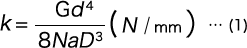However, to calculate the load, it is necessary to consider the initial tension. If this initial tension is Pi, any load (P) will be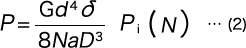From formula (1), the deflection (δ) is as per the following formula.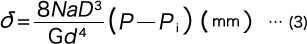The shear stress τ0・τ are calculated with the following formulas just like with the compression coil spring.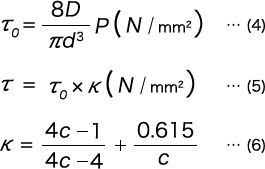### Stress of the Hook Part of Extension Springs

As the tensile stress and the shear stress based on the bending moment and the torsion moment are generated from the hook part, it is complicated to reach an accurate calculation. Here, we introduce approximate calculations for the widely used machine half hooks and U-shaped hooks.

#### （i）In the Case of a Machine Half Hook

In Figure 1, the maximum tensile stress value occurs inside part A, and the maximum shear stress value occurs inside part B.
As the maximum tensile stress inside part A is the sum of the bending moment (M) and the tensile stress according to the axial load (P), it becomes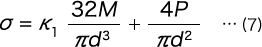Here, K1 is the stress concentration coefficient based on the curvature, and if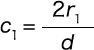, it can be found with the following formula.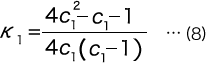When formula (7) is rearranged, we get the following formula.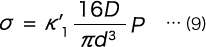K1, however, is as follows.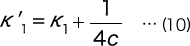Here, C is the spring index of the coil part.
The maximum shear stress at the inside of part B is due to the torsion moment M.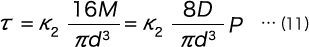Here, Ｋ2 is the stress concentration coefficient based on the curvature, and if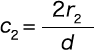, it can be found with the following formula.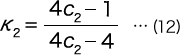#### （ii）In the Case of an U-shaped Hook

In Figure 2, the maximum tensile stress value occurs inside part A, and the maximum shear stress value occurs inside part B.
As the maximum tensile stress inside part A is the sum of the bending moment (M) and the tensile stress according to the axial load (P), it becomes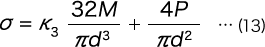Here, Ｋ3 is the stress concentration coefficient based on the curvature, and if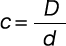, it can be found with the following formula.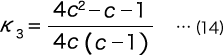When formula (13) is rearranged, we get the following formula.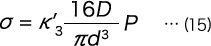K’3, however, is as follows.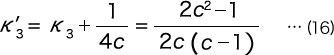The maximum shear stress at part B is given with formula (11) just like in the case of the machine half hook. Hooks of other shapes may also be considered in the same way.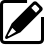Product information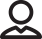Our Proposals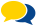Popular questions